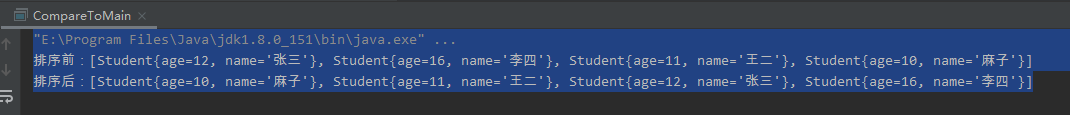2020-07-14 15:15

# 高分悬赏：Java语言求高人编写一个完整的调用的例子，利用compareTo来实现快速排序

• 写回答
• 关注问题
• 收藏
• 邀请回答

#### 2条回答默认 最新

•毕小宝 2020-08-07 13:44
已采纳

参考代码：

``````//一趟快速排序的过程
public static int procedure(Object[] arr,int start,int end){
Object flag = arr[start];   //上述文字中描述的枢轴记录，通常是第一个记录即arr[start]
Object temp = null;
//start =end 时，一趟快速排序结束
while(start < end){
while(start < end && arr[end].hashCode() >= flag.hashCode()){
end--;
}
temp = arr[start];
arr[start] = arr[end];
arr[end] = temp;
while(start < end && arr[start].hashCode() <= flag.hashCode()){
start++;
}
temp = arr[start];
arr[start] = arr[end];
arr[end] = temp;
}
//返回start值，start值为整个数组前半区和后半区的分隔坐标
return start;
}

``````
打赏 评论
•zsc我行我素 2020-07-14 15:41
``````import java.util.ArrayList;
import java.util.Collections;
import java.util.List;

public class CompareToMain {

public static void main(String[] args) {
List<Student> list = new ArrayList<>();
System.out.println("排序前："+ list);
// java.lang.util包中提供的排序算法，会调用Student对象的compareTo方法进行对象大小比较，compareTo方法中可以自定义大小比较规则，比如按年龄排序
// 该方法传入list中的对象必需实现comparable接口的compareTo方法
Collections.sort(list);
System.out.println("排序后："+ list);
}
}
``````
``````public class Student implements Comparable<Student> {

private int age;

private String name;

public Student(int age, String name) {
this.age = age;
this.name = name;
}

@Override
public int compareTo(Student s) {
// 这里可以自定义比较大小规则，比如按年龄
if (s.getAge() > age) {
return -1;
} else {
return 1;
}
}

@Override
public String toString() {
return "Student{" +
"age=" + age +
", name='" + name + '\'' +
'}';
}

///////////////////////////////////getter and setter///////////////////////////////

public int getAge() {
return age;
}

public void setAge(int age) {
this.age = age;
}

public String getName() {
return name;
}

public void setName(String name) {
this.name = name;
}
}
``````

执行结果：compareTo方法主要用来自定义对象的比较大小规则

打赏 评论